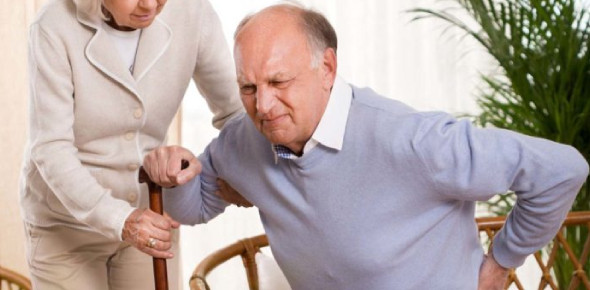# Problems On Ages - Aptitude Questions And Answers :Quiz

7 Questions | Attempts: 2162SettingsThis quiz is designed to determine your ability in a particular skill or field of knowledge. The questions below test whether you can give the correct ages for the people below depending on how you understand them. Give it a try, as it will increase your brain activity. Good luck!

• 1.
In three more years, Miguel's grandfather will be six times as old as Miguel was last year. When Miguel's present age is added to his grandfather's present age, the total is 68. How old is each one now?
• A.

The grandfather is 57 years old Miguel is presently 11 years old

• B.

The grandfather is 45 years old Miguel is presently 23 years old

• C.

The grandfather is 60 years old Miguel is presently 8 years old

• D.

The grandfather is 55 years old Miguel is presently 13 years old

• E.

The grandfather is 48 years old Miguel is presently 20 years old

• 2.
One-half of Heather's age two years from now plus one-third of her age three years ago is twenty years. How old is she now?
• A.

Heather is 36 years old.

• B.

Heather is 45 years old.

• C.

Heather is 67 years old.

• D.

Heather is 24 years old.

• E.

Heather is 56 years old.

• 3.
John is twice as old as his friend Peter. Peter is 5 years older than Alice. In 5 years, John will be three times as old as Alice. How old is Peter now?
• A.

Peter is now 10 years old.

• B.

Peter is now 5 years old.

• C.

Peter is now 45 years old.

• D.

Peter is now 21 years old.

• E.

Peter is now 3 years old.

• 4.
The sum of Ben and carol's age is 36. Nine years ago, Ben was twice as old as Carol. How old is each now?
• A.

Ben's age now is 19, Carol's age now is 17.

• B.

Ben's age now is 18, Carol's age now is 18.

• C.

Ben's age now is 21, Carol's age now is 15.

• D.

Ben's age now is 31, Carol's age now is 5.

• E.

Ben's age now is 23, Carol's age now is 13.

• 5.
Sam is 3 years younger than Alex and Alex is 7 years older than Mary. If the sum of their ages is 44, find each persons age.
• A.

Alex's age = 18 Sam's age = 15 Mary's age = 11

• B.

Alex's age = 18 Sam's age = 15 Mary's age = 11

• C.

Alex's age = 18 Sam's age = 15 Mary's age = 11

• D.

Alex's age = 18 Sam's age = 15 Mary's age = 11

• E.

Alex's age = 12 Sam's age = 14 Mary's age = 1

• 6.
Miguel is 46 years old. He is 4 years older than thrice his Niko's age. Find the age of Niko.
• A.

Niko is 15 years old.

• B.

Niko is 14 years old.

• C.

Niko is 13 years old.

• D.

Niko is 10 years old.

• E.

Niko is 11 years old.

• 7.
Edward, Bryan and Mark are cousins.Edawrd’s age is one-third of Bryan and Mark is five years elder than Bryan. If the sum of the age of the cousins is 40, find the ages of each.
• A.

Edward=5 Bryan=15 Mark=20

• B.

Edward=2 Bryan=13 Mark=25

• C.

Edward=9 Bryan=14 Mark=17

• D.

Edward=11 Bryan=13 Mark=16

• E.

Edward=10 Bryan=8 Mark=22

## Related TopicsBack to top
×

Wait!
Here's an interesting quiz for you.